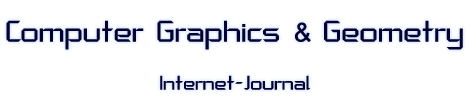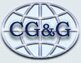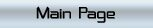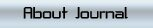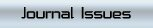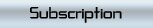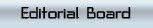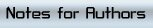### COMPUTER GRAPHICS & GEOMETRY

Issue Year: 1999
Date: Winter
Volume: 1
Number: 3
Pages: 1-24

 Article Name: CLUSTERING FOR DATA REDUCTION AND APPROXIMATION Author: T. Schreiber Address: T. Schreiber University of Kaiserslautern, Germany Abstract: This paper describes a solution to the following problem: Given a large set of arbitrarily distributed and weighted data points, find a much smaller set of cluster center points, which minimizes the sum of the squared distances between each data point and the nearest cluster center point. The weight of each data point may be chosen by the user depending on the importance or correctness of that point. An efficient clustering algorithm using local procedures on Voronoi diagrams is introduced. At the same time the method produces hierarchical Delaunay triangulations of the data points at different degrees of accuracy.

Issue contents